# Workday Excel Function

Many professionals use Excel in their daily activities. Some include accountants, managers, project managers, financial analysts, data scientists and analysts, data journalists, business analysts, e.t.c. Excel provides them with different functions that perform different tasks. They help the professionals simplify complex tasks, do jobs faster, automate repetitive tasks, and be exceptional with their team. Knowing how to use the functions well separates you from the rest of Excel users who use long formulas instead of embracing functions. The article will cover more about the Workday Excel function, a data time function that automates most date calculations.

## What Is a Workday Function?

A workday function is an Excel function that provides users with a date that shows the number of working days before or at the starting date. Users can also use the function to subtract or add any days from a given date. When calculating analysis in finance using the workday function, you have to eliminate all the weekends and holidays when calculating the invoice dates, the expected delivery date, and the number of days there was work.

The formula for calculating the workday function is:

= WORKDAY(start_date, days, [holidays])

It has three arguments.

• The start date represents the date you started.
• Days-It is the number of days you need to add to the start date. Most of them consist of non-holidays and non-weekend days. When you get a positive value, it shows a future date, while when you get a negative value, it shows the date in the past.
• Holidays-It is optional. It normally starts for days that you did not report to the job. Most of the days are holidays. You can use cells with the date or serial numbers that represent the date.
For example:

We have the starting dates as 12/08/2022, 13/08/2022, 14/08/2022, and 15/08/2022 and the number of days as 32,33,34,35, respectively. To calculate the output, we use the following formulas for each starting day as follows: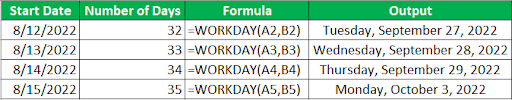### When Do We Use The Excel Workday Function?

We use the Excel Workday function when we need to do the following:

• When we want to subtract or add any dates from a specific date.
• When you want to generate different random dates between two dates.
• When you want to know the days, you will report to work. (workdays)
• When you want to calculate when you can complete a particular project.
• When you want to know the working days and holidays in a certain period.

Become a Workday Certified professional by learning this HKR Workday Training !

### Workday Training

• Lifetime LMS & Faculty Access
• Real-world & Project Based Learning

### How To Use The Workday Function In Excel?

Let us look at several examples of how you can use the function to do different calculations in excel.

Example 1

In our first example, let us assume we have the following start dates and number of working days. We shall use the following formula as shown in the excel sheet.

Start dates  Number of working days

10/08/2022   3

13/08/2022 7

18/08/2022 23

24/08/2022 10

Copy the start dates into the A column excel sheet and the number of working days into column B. Create another column under C and name it Formula, and the last column name it Result. Let us now calculate the first value, the date will be under A2, and the number of working days will be B2, the formula will be =WORKDAY(A2, B2) which will bring the result. The others will use the same formulas as shown below.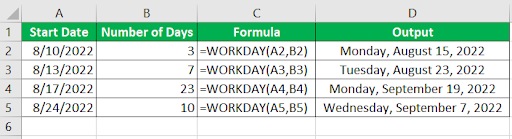Example 2

In this example, we will look at a case where a particular team has to work on a specific project, and the team has assumed it will take 40 days. The team does not work on holidays and weekends. Let us calculate when they will finish the project.

Let's assume they will start on 20/08/2022. Let us place our starting date in cell B1, the number of days in B2, and the holidays will be in cells D and E. To calculate the day the team will finish the project, we will use the WORKDAY function as shown below:

=WORKDAY(B1,B2,E2:E11)

The E2 and E11 represent the holidays.

The result can be calculated in Excel as shown below: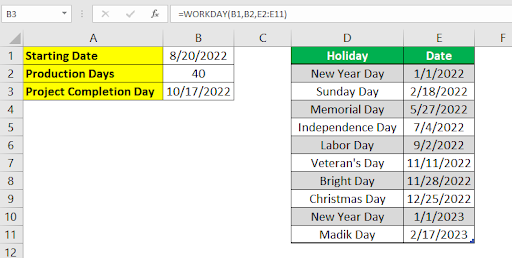Example 3
In this example, we have one date. We want to use the date to calculate the coming days while skipping the non-working days. Our starting date will be 2/19/2022, and we will apply the Workday function such that it adds 1 while ignoring the nonworking days. Since our values are in cell A. The formulae will be as follows:

=WORKDAY(A2,1), and you apply it to the rest of the cells upto where you want. You will notice that the formula has skipped all the weekends when the team is not working.

The excel sheet will look like this: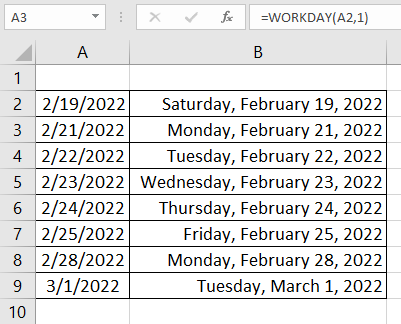Want to know more about Workday,visit here Workday Tutorial !Example 4

In this example, we have working days in a column. We want to confirm whether, among the days, there are non-working days. We want to have two outputs where when it is a working day, it returns working, and when it is a holiday, it returns a holiday. We will use the following formula:

=IF(WORKDAY(A2-1,1,\$E\$3:\$E\$4)=A2,”Working”,”Holiday”)

The excel sheet will be as follows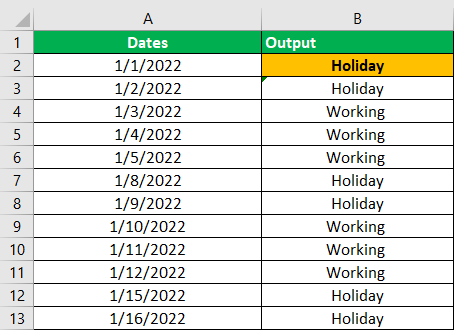Example 5

In this example, we will use the workday function to calculate the next business day that will come after six months. We use the following formulae

=WORKDAY(EDATE(A2,6)-1,1,A8:A10)

The formula gets the next six months. EDATE handles the future months and subtracts 1 from the formulae, assigning the results to the start_date. Here is the excel sheet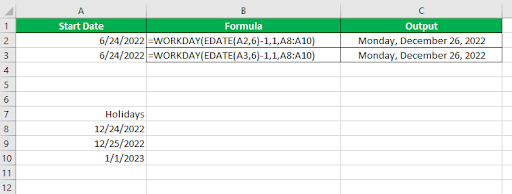Example 6

How to calculate several random days when given two dates? The random days should exclude non-working days, which are weekends. Our starting date will be 1/31/2022, and the ending date will be 5/25/2022. To get the result, we will introduce the RANDBETWEEN concept, which will help calculate the days. The formulae used will be as shown below:

=C4=WORKDAY(RANDBETWEEN(\$B\$2,\$B\$3)-A22,1)

Cell C will have the random days, and B will have the start and end dates. In our formula, we subtract 2 to avoid getting the random date that is the same as the end date. When you apply the random formulae, you get the following dates as shown in the sheet below.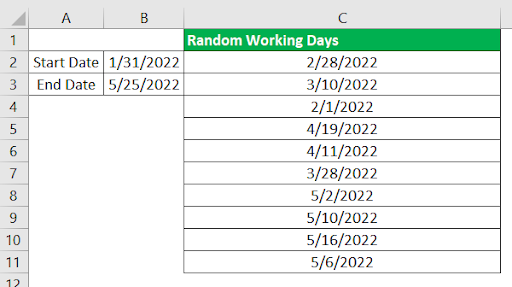Top 30 frequently asked Workday Interview Questions !

Explore Workday payroll Functional Sample Resumes Download & Edit, Get Noticed by Top Employers!

### Workday Training

Weekday / Weekend Batches

#### What Are Workday Function Errors?

Some of the common Workday function errors include the following:

• #NUM! - The error is common when the start-date is given when you add it to the supplied days, you get an error that says invalid date.
• #VALUE! - The error normally occurs when the number of days used or supplied by the user is non-numeric. It can also happen when the start_date or the number of days or values given under the holiday is invalid.

#### Important Characteristics Of Workday Function

Some of the important characteristics of workday function include the following:

• When you use a start_date and invalid days, you are likely to get a #NUM error.
• If you use the third argument(holiday), you must ensure that if it is a range, you pass it as an absolute value as its value may change when applying the formula to other cells.
• Under the day's argument, if you use a value that is not an integer. The function truncates the integer into the nearest value. For example, a value like 20.6 gets truncated to 20.
• When you input the data values using double quotes, sometimes misinterpret them. Instead of using quotes to enter dates as text values, you can use the date function. It makes excel understand the date values.
• If the date you use in your project has time, the function takes the date value and assumes the time part.
• You can enter the date value in several ways: by getting it from another function, having a date in text form, and surrounding your date with double quotes.
• If the values you used in the start_date or the days arguments are invalid, or the value under the days is not numerical, you will likely get a VALUE error.
###### Conclusion

Excel has many functions that make calculations fast and easier. The workday function is one of the date and time functions that has helped solve many calculations involving dates. Many Excel users like data analysts, accountants, and students must understand how to use the excel functions in their daily activities to make their work easier. You can learn these functions on your own or attend workday training that will equip you with the required skills that match the job specifications and boost your career.

Related blogs:

#### Find our upcoming Workday Training Online Classes

• Batch starts on 5th Apr 2023, Weekday batch

• Batch starts on 9th Apr 2023, Weekend batch

• Batch starts on 13th Apr 2023, Weekday batch